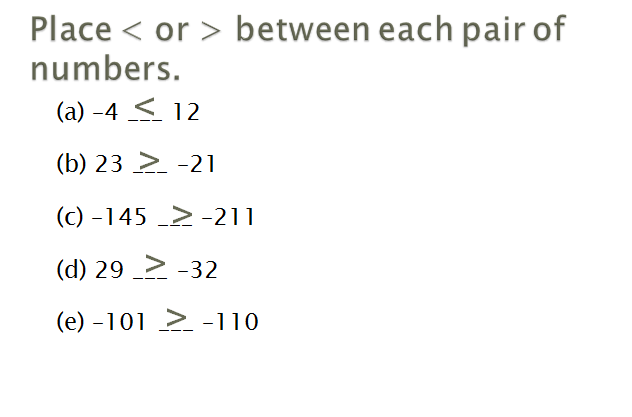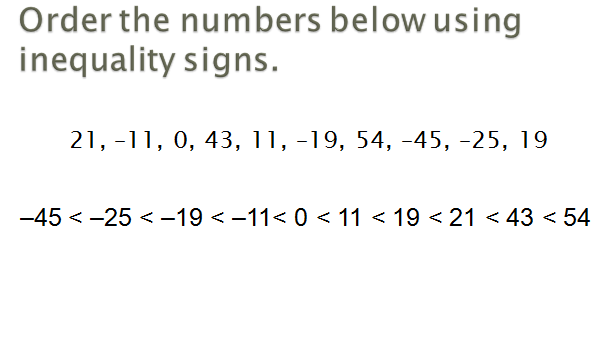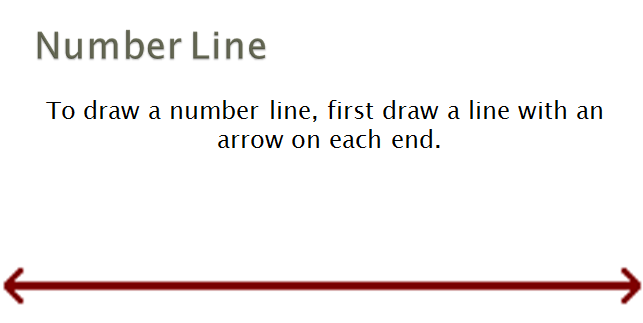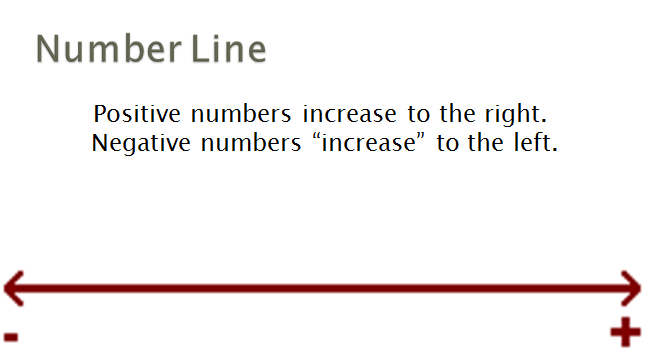# SCC Education

## Inequalities: An Introduction

Inequalities: An Introduction
An inequality is like an equation, but instead of an equal sign (=) it has one of these signs:
<   :  less than
:  less than or equal to
>  :  greater than

:  greater than or equal to